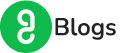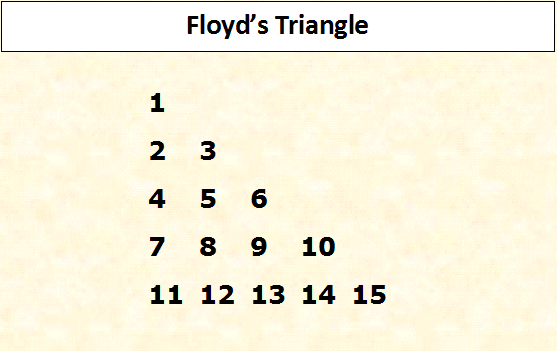# Top 10 Java Programs For Freshers | Start Coding With A Flair!

Hey, I found you searching for the top 10 Java Programs for freshers.

Welcome to GUVI Blogs.

This is Prashanth, a Java and Selenium Expert, sharing the experience that has taken me a long way! Learn, Code, & Practice here with me!

## Why refer to this list of ‘top 10 Java Programs for freshers’?

This is a list of 10 Java coding interview questions and answers. The below array of questions is a good place to get started and prepare before you appear for any Java Programming interviews.

First of all, I would like to tell you that Java Interview questions are mostly based on programming, logical analysis, and problem-solving skills. It is always better to get it right in the first place. Well, obviously you may be able to solve and find answers to these Java programming questions by yourself.

However, if you find yourself stuck at some place and end up Googling it, then it becomes a larger challenge! This is to say that, in Google, you will find ‘n’ number of alternative solutions to the same problem. Although, knowing more than one way to solve any programming question or coding problem in Java is a factor that impresses the interviewer. However, most of the time it becomes overwhelming and too confusing to take.So, I have specially curated a list of the top 10 Java programs. This list mainly contains basic programs asked in Interviews. Let’s get started!

### Verify a number is Even/Odd

This is one of the most commonly asked coding questions. Find the easiest solution below.

```import java.util.Scanner;

public class EvenOdd{
public static void main(String[] args){
Scanner in = new Scanner(System.in);
System.out.println("Enter a number which you want to check whether that is even or odd");
int n = in.nextInt();

if(n%2==0){
System.out.println(n+" is an even number.");
}else{
System.out.println(n+" is an odd number.");

}
}
}
```

```Enter a number which you want to check whether that is even or odd
4
4 is an even number.```

### Swapping Numbers without using the 3rd variable

```import java.util.Scanner;

public class Swapping{
public static void main(String[] args){
Scanner in = new Scanner(System.in);
System.out.println("Enter the 1st number: ");
int x = in.nextInt();
System.out.println("Enter the 2nd number: ");
int y = in.nextInt();

System.out.println("Initial value of x: "+x+" and y: "+y);

x = x+y;
y = x-y;
x = x-y;

System.out.println("After swapping value of x: "+x+" and y: "+y);
}
}
```

### Output

```Enter the 1st number:
43
Enter the 2nd number:
56
Initial value of x: 43 and y: 56
After swapping value of x: 56 and y: 43
```

### Factorial of a number

```import java.util.Scanner;

public class Factorial{
public static void main(String[] args){
Scanner in = new Scanner(System.in);
System.out.println("Enter the number whose factorial you want: ");
int n = in.nextInt();
int f =1;
for(int i=n; i>0; i--){
f = f*i;
}
System.out.println("Factorial of "+n+" is "+f);
}
}
```

### Output

```Enter the number whose factorial you want:
6
Factorial of 6 is 720
```

[maxbutton id=”3″]

### How to get the prime numbers between a given range

```package javaTutorial;

import java.util.ArrayList;
import java.util.Scanner;

public static void main(String[] args){
Scanner in = new Scanner(System.in);
System.out.println("Enter a number from which you want prime number: ");
int p1 = in.nextInt();
System.out.println("Enter one more number till which you want prime number: ");
int p2 = in.nextInt();
ArrayList<Integer> prime = new ArrayList<Integer>();
int i=2;
for(int p=p1; p<=p2; p++){
i=2;
for(; i<10; i++){
if(p%i==0 && p!=i){
break;
}
}
if(i==10){
}
}
System.out.println("Prime numbers between "+p1+" and "+p2+" are: ");
for(int j=0; j<prime.size(); j++){
System.out.print(prime.get(j).toString()+", ");
}
}
}
```

### Output

```Enter a number from which you want prime number:
10
Enter one more number till which you want prime number:
30
Prime numbers between 10 and 30 are:
11, 13, 17, 19, 23, 29,
```

### Check a number is prime or not.

Note- A number is prime if it is not divisible by any other number except itself.

```import java.util.Scanner;

public static void main(String[] args){
Scanner in = new Scanner(System.in);
System.out.println("Enter a number greater than 2 which you want to check whether that number is prime or not: ");
int p = in.nextInt();
int i=2;
for(; i<10; i++){
if(p%i==0 && p!=i){
System.out.println("Entered number "+p+" is not a prime number.");
break;
}
}
if(i==10){
System.out.println("Entered number "+p+" is a prime number.");
}
}
}
```

### Output

```Enter a number greater than 2 which you want to check whether that number is prime or not:
139
Entered number 139 is a prime number.
```

### Check if a number is Armstrong or not.

Note- A number is Armstrong if the sum of the cubes of digit of the number is equal to the number. ex- 407 = 4*4*4 + 0*0*0 + 7*7*7

```import java.util.Scanner;

public class ArmstrongNum{
public static void main(String[] args){
Scanner in = new Scanner(System.in);
System.out.println("Enter a number which you want to check whether that is armstrong or not: ");
int n = in.nextInt();
int a = n, r=0, s=0;

while(a!=0){
r = a%10;
a = a/10;
s = s + r*r*r;
}
if(s==n){
System.out.println("Number "+n+" is an armstrong number.");
}else{
System.out.println("Number "+n+" is not an armstrong number.");
}
}
}
```

### Output

```Enter a number which you want to check whether that is armstrong or not:
407
Number 407 is an armstrong number.
```

[maxbutton id=”3″]

### Floyd Triangle

Note- Floyd Triangle is like 1 2 3 4 5 6 7 8 9 10 ———— Code-

```import java.util.Scanner;

public class FloydTriangle{
public static void main(String[] args){
Scanner in = new Scanner(System.in);
System.out.println("Enter the number of rows which you want in your Floyd Triangle: ");
int r = in.nextInt();
int n=0;
for(int i=0; i<r; i++){
for(int j=0; j<=i; j++){
System.out.print(++n+" ");
}
System.out.println();
}
}
}
```

### Output

```Enter the number of rows which you want in your Floyd Triangle:
5
1
2 3
4 5 6
7 8 9 10
11 12 13 14 15```

### Palindrome of String or reverse a String.

```import java.util.Scanner;

public class PalindromeString{
public static void main(String[] args){
Scanner in = new Scanner(System.in);
System.out.println("Enter the string which you want to check whether that is palindrome or not: ");
String s = in.next();
String r = "";
for(int i=s.length()-1; i>=0; i--){
r = r+s.charAt(i);
}
System.out.println("Reverse of entered string "+s+" is "+r);
if(r.equals(s)){
System.out.println("String "+s+" is palindrome.");
}else{
System.out.println("String "+s+" is not palindrome.");
}
}
}
```

### Output

```Enter the string which you want to check whether that is palindrome or not:
selenium
Reverse of entered string selenium is muineles
String selenium is not palindrome.
```

### Binary Search

```import java.util.Arrays;
import java.util.Scanner;

public class BinarySearch{
public static void main(String[] args){
Scanner in = new Scanner(System.in);
System.out.println("Enter the size of the array which should be greater than zero else it will throw InputMismatchException : ");
int size = in.nextInt();
int[] array = new int[size];
System.out.println("Enter the elements of the array: ");
for(int i=0; i<size; i++){
array[i] = in.nextInt();
}
System.out.println("Enter the search element: ");
int s = in.nextInt();

Arrays.sort(array); //binary search will work on sorted array only so sort first
int first, last, middle;
first=0;
last = size-1;
middle = (first+last)/2;
int i=0;
for(; i<size; i++){
if(s>array[middle]){
first = middle+1;
}else if(s<array[middle]){
last = middle-1;
}else{
printArray(array);
System.out.println("Element "+s+" found in the array.");
break;
}
middle= (first+last)/2;
}
if(i==size){
printArray(array);
}
}
public static void printArray(int[] a){
System.out.println("Array of elements: ");
System.out.print("{");
for(int i:a){
System.out.print(i+",");
}
System.out.println("}");
}
}
```

### Bubble Sort – last but not least in the list of top 10 Java Programs for freshers

```package SeleniumMakeItEasy;

import org.apache.commons.lang3.ArrayUtils;

public class BubbleSort{
public static void main(String[] args){
int[] a = {2,3,2,5,3,3,6,1,2,5};
int l = a.length;

for(int i=0;i<l; i++){

for(int j=0; j<l-1; j++){
if(a[j]>a[j+1]){
a[j] = a[j] + a[j+1];
a[j+1] = a[j] - a[j+1];
a[j] = a[j] - a[j+1];
}else if(a[j]==a[j+1]){
a = ArrayUtils.remove(a,j);
l = a.length;
}
}
}
for(int s: a){
System.out.println(s);
}

}
}
[maxbutton id="3"]```

Check out our Project Ideas – Here

Looking out for more such programs and source codes? Why don’t you leave us your contact details and we will get back to you shortly?

### Related Posts

How Guvi helped a First-Year student get placed in Juspay

10 things college students can do in their vacation to improve Job prospects.

How to succeed in Campus Interviews (Important Tips)

Contact Form

By clicking 'Submit' you Agree to Guvi Terms & Conditions.

Our Trending Courses

Our Learners Work at

## Author Bio## Our Live Classes

### Full Stack Development Program (FSD)

Learn Javascript, HTML, CSS, Java, Data Structure, MongoDB & more

### IIT-M Advanced Programming & Data Science Program

Learn Python, Machine Learning, NLP, Tableau, PowerBI & more

### Automation and Testing Program

Learn Selenium, Python, Java, Jenkins, Jmeter, API Testing & more

### UI/UX Program

UX Processes, Design systems, Responsive UI, & more with placement assistance.

## Related Articles

If you are reading “var functionName = function and function functionName,” we can understand how confusing it is for you.